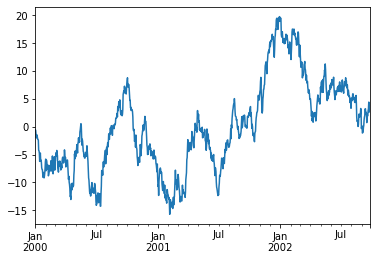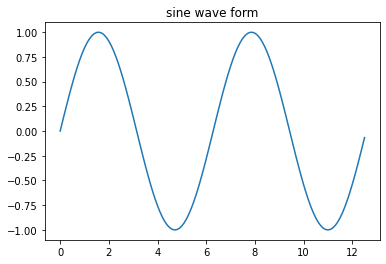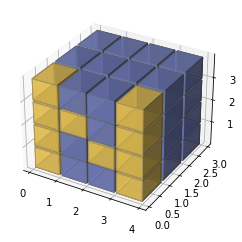# 必备工具包

## Numpy

NumPy(Numerical Python) 是 Python 语言的一个扩展程序库，支持大量的维度数组与矩阵运算，此外也针对数组运算提供大量的数学函数库。

• 一个强大的 N 维数组对象 ndarray

• 整合 C/C++/Fortran 代码的工具

• 线性代数、傅里叶变换、随机数生成等功能

ps: 说道这个 N-Array 不得不说成也萧何败萧何。java 为了避免程序的复杂性，本身是不支持 N-Array 的，所以原来使用 DL4j 进行机器学习的时候就会觉得有些别扭。

## Pandas

Pandas 是一个开源的，BSD许可的库，为Python编程语言提供高性能，易于使用的数据结构和数据分析工具。

Pandas 的主要数据结构是 Series（一维数据）与 DataFrame（二维数据），这两种数据结构足以处理金融、统计、社会科学、工程等领域里的大多数典型用例

ps: 一般这个工具在债券、股票分析领域是非常常见的。

## Matplotlib

Matplotlib 是一个综合库，用于在Python中创建静态，动画和交互式可视化。

## Seaborn

Seaborn 是基于matplotlib的Python数据可视化库。

# Numpy 入门

## 引入

import numpy as np


## 入门

import numpy as np
print (np.std([1,2,3,4]))


1.118033988749895


# Pandas 入门

## 引入

import numpy as np
import pandas as pd


## 十分钟入门

### 生成对象

import numpy as np
import pandas as pd

s = pd.Series([1, 3, 5, np.nan, 6, 8])
s


0    1.0
1    3.0
2    5.0
3    NaN
4    6.0
5    8.0
dtype: float64


### 可视化

import pandas as pd

ts = pd.Series(np.random.randn(1000), index=pd.date_range('1/1/2000', periods=1000))
ts = ts.cumsum()
ts.plot()# Matplotlib 入门

## 引入

import numpy as np
import matplotlib.pyplot as plt


## 绘制正弦曲线

# 计算正弦曲线上点的 x 和 y 坐标
x = np.arange(0,  4  * np.pi,  0.1)
y = np.sin(x)
plt.title("sine wave form")
# 使用 matplotlib 来绘制点
plt.plot(x, y)
plt.show()## 更进一步

import matplotlib.pyplot as plt
import numpy as np

def explode(data):
size = np.array(data.shape)*2
data_e = np.zeros(size - 1, dtype=data.dtype)
data_e[::2, ::2, ::2] = data
return data_e

# build up the numpy logo
n_voxels = np.zeros((4, 3, 4), dtype=bool)
n_voxels[0, 0, :] = True
n_voxels[-1, 0, :] = True
n_voxels[1, 0, 2] = True
n_voxels[2, 0, 1] = True
facecolors = np.where(n_voxels, '#FFD65DC0', '#7A88CCC0')
edgecolors = np.where(n_voxels, '#BFAB6E', '#7D84A6')
filled = np.ones(n_voxels.shape)

# upscale the above voxel image, leaving gaps
filled_2 = explode(filled)
fcolors_2 = explode(facecolors)
ecolors_2 = explode(edgecolors)

# Shrink the gaps
x, y, z = np.indices(np.array(filled_2.shape) + 1).astype(float) // 2
x[0::2, :, :] += 0.05
y[:, 0::2, :] += 0.05
z[:, :, 0::2] += 0.05
x[1::2, :, :] += 0.95
y[:, 1::2, :] += 0.95
z[:, :, 1::2] += 0.95

ax.voxels(x, y, z, filled_2, facecolors=fcolors_2, edgecolors=ecolors_2)

plt.show()（1）数据的初始化

（2）数据的计算、分析

（3）数据的可视化

NumPy 教程

Pandas 中文教程